Published: 15 May 2019

# Variable learning rate EASI-based adaptive blind source separation in situation of nonstationary source and linear time-varying systems

Cheng Wang1
Haiyang Huang2
Yiwen Zhang3
Yewang Chen4
1State Key Laboratory for Strength and Vibration of Mechanical Structures, Xi’an Jiaotong University, 710049, Xi’an, P. R. China
1, 2, 3, 4College of Computer Science and Technology, Huaqiao University, 361021, Xiamen, P. R. China
Corresponding Author:
Cheng Wang
Views 177

#### Abstract

In the case of multiple nonstationary independent source signals and linear instantaneous time-varying mixing systems, it is difficult to adaptively separate the multiple source signals. Therefore, the adaptive blind source separation (BSS) problem is firstly formally expressed and compared with tradition BSS problem. Then, we propose an adaptive blind identification and separation method based on the variable learning rate equivariant adaptive source separation via independence (EASI) algorithm. Furthermore, we analyze the scope and conditions of variable-learning rate EASI algorithm. The adaptive BSS simulation results also show that the variable learning rate EASI algorithm provides better separation effect than the fixed learning rate EASI and recursive least-squares algorithms.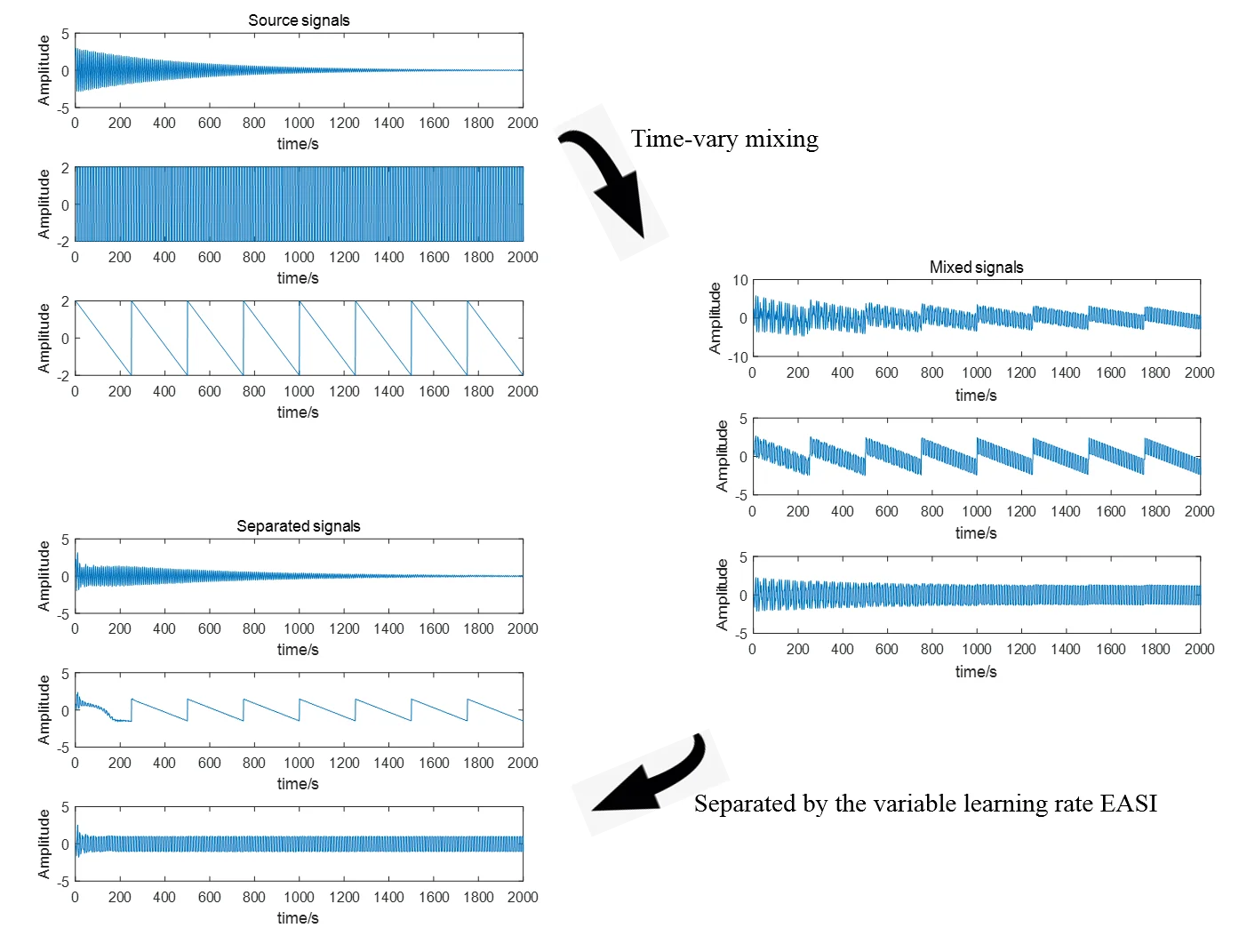#### Highlights

• Online real-time BSS problems are formally expressed and compared to traditional BSS issues.
• This paper applies a variable learning rate EASI algorithm to the adaptive BSS problem.
• The similarity coefficient and Vestigial Quadratic Mismatch (VQM) are used as quantitative evaluation indicators of the waveform similarity of the separated source signals.
• A simulation is designed to verify the correctness of our approach. The fixed learning rate EASI and RLS algorithms are used for comparison.

## 1. Introduction

There is an increasing demand for dynamic systems to be safer and more reliable . In mechanical fault diagnosis, the extraction of fault characteristic information is indispensable , although the complexity of mechanical devices means that mechanical vibration signals usually have mixed and multipath effects. Furthermore, there may be some frequency superposition in the signals, so it is challenging to extract feature information. When multiple signals are statistically independent, the technique of blind source separation (BSS) can identify the original signals through the use of sensors, regardless of the location information, signal quantity, and so on .

Recently, BSS has attracted considerable attention. It has been widely used in many practical applications, such as telecommunications , biomedical signal processing , vibration source enumeration and identification , image processing , voice signal classification , fault diagnosis , and structural health monitoring [10, 11]. The earliest application of BSS was in the failure analysis of a gearbox . BSS can be conducted through two popular approaches, namely second-order blind identification  and independent component analysis (ICA) . Traditional BSS methods have two main disadvantages : they operate offline, and require the entire signal to perform separation and identification. The use of offline BSS methods for online problems is time-consuming and inefficient.

The first adaptive form of BSS was proposed by Jutten and Herault . Cardoso and Laheld developed a different adaptive approach , using the ‘relative gradient’ adaptive algorithm based on serial updating, whereby the separating matrix is updated in each step when a new sample is received. These adaptive algorithms are known as equivariant adaptive source separation via independence (EASI). However, because of their constant learning rate (step-size), typical EASI algorithms face a trade-off between stability and convergence speed .

Methods with adaptive learning rate have been widely studied, as the variable learning rate makes the algorithm effective in non-stationary environments. Xie et al.  adjusted the learning rate using mutual information, and Zhang et al.  changed the learning rate by estimating a performance index. For time-varying mixing channels, the variable learning rate sign natural gradient algorithm was proposed by Yuan et al. . Shifeng et al.  developed a variable leaning rate composed of two adaptive separation systems, while Gao et al.  designed a performance-index-based EASI method for direct sequence code division multiple access (DS-CDMA) systems. Chambers et al. presented a method for dealing with abrupt changes in the mixing matrix , and an adaptive algorithm that can separate noisy time varying mixtures has been reported by Enescu et al. . DeYoung et al.  applied BSS techniques to mixtures of digital communication signals in which the sources are mobile or the environment is changing, and the mixing matrix will vary with time. Their results indicate that the main difficulty in the separation phase is the ill-conditioned nature of the channel matrix. Chen et al.  used a retrospective online EASI method to deal with the problem of sudden changes in the time-varying environment. A time-varying mixing matrix with stationary source signals was investigated by Bulek et al. . In an earlier article, we proposed two algorithms (recursive least-squares (RLS) and Recursive-EASI)  to solve the problem of time-varying source signals and time-varying systems simultaneously.

However, all of the above research work did not describe the adaptive BSS problem in detail. Furthermore, these previous methods focus on algorithm performance and do not evaluate the separation results. In this paper, we formally describe the adaptive BSS problem and propose the use of a variable learning rate EASI-based method to solve the problem of nonstationary source signals in a different slowly time-varying environment. Compared with our earlier algorithms , the variable learning rate EASI method described in this paper is totally different (R-EASI selects a reference point, and the method proposed in this paper uses a variable learning rate), and we present a detailed theoretical derivation. In the simulation section, we describe the parameter settings, evaluation index, and simulation analysis.

The primary contributions of this paper can be summarized as follows:

1) The problem of online real-time BSS under a time-varying system and nonstationary source signals is formally expressed and compared with the traditional BSS problem. The differences between the two problem types are explained in detail.

2) This paper applies a variable learning rate EASI algorithm for the problem of adaptive BSS for a mixing matrix that varies slowly with time and a nonstationary environment. In addition, the scope and conditions of the method are identified.

3) The similarity coefficient and Vestigial Quadratic Mismatch (VQM) are used as quantitative evaluation indexes of the waveform similarity of the separated source signals.

4) We design a simulation to verify the correctness of our method. The fixed learning rate EASI and RLS algorithms are used for comparison.

The remainder of this article is arranged as follows. Section 2 introduces the model and the concept of adaptive BSS. Section 3 describes the process of our EASI method in detail. Section 4 presents the simulation verification procedure and results. Finally, we state the conclusions to this study and ideas for future research in Section 5.

## 2. Adaptive BSS model for linear instantaneous mixing systems and nonstationary source signals

### 2.1. Description of adaptive BSS

In traditional BSS, the mixing matrix $\mathbf{A}$ is constant. However, in reality, the way in which signals mix varies with time. We call the linear instantaneous mixing matrix ${\mathbf{A}}_{t}$. The model can be described as [25, 27, 28]:

1
$\mathbf{X}\left(t\right)={\mathbf{A}}_{t}\mathbf{S}\left(t\right)+\mathbf{N}\left(t\right),$

where $\mathbf{X}\left(t\right)=\left[{\stackrel{\to }{x}}_{1}\left(t\right),{\stackrel{\to }{x}}_{2}\left(t\right),...,{\stackrel{\to }{x}}_{m}\left(t\right){\right]}^{T}\in {R}^{m×L}$ denotes the observed signals, ${\mathbf{A}}_{t}\in {R}^{m×k}$, and $\mathbf{N}\left(t\right)\in {R}^{m×L}$ denotes the observation noise. $\mathbf{S}\left(t\right)=\left[{\stackrel{\to }{s}}_{1}\left(t\right),{\stackrel{\to }{s}}_{2}\left(t\right),...,{\stackrel{\to }{s}}_{k}\left(t\right){\right]}^{T}\in {R}^{k×L}$ represents the nonstationary source signals. Without considering any observation noise:

2
$\mathbf{X}\left(t\right)={\mathbf{A}}_{t}\mathbf{S}\left(t\right).$

In our setting, the separation matrix ${\mathbf{B}}_{t}\in {R}^{k×m}$ is unknown but should vary with time, and the separated multiple nonstationary independent signals $\mathbit{Y}\left(t\right)=\left[{\stackrel{\to }{y}}_{1}\left(t\right),{\stackrel{\to }{y}}_{2}\left(t\right),...,{\stackrel{\to }{y}}_{k}\left(t\right){\right]}^{T}\in {R}^{k×L}$ can be got by:

3
$\mathbf{Y}\left(t\right)={\mathbf{B}}_{t}\mathbf{X}\left(t\right),$

and the separated signals latest value $\stackrel{\to }{y}\left(t\right)={\mathbf{B}}_{t}\stackrel{\to }{x}\left(t\right)={\mathbf{B}}_{t}{\mathbf{A}}_{t}\stackrel{\to }{s}\left(t\right)={\mathbf{E}}_{t}\stackrel{\to }{s}\left(t\right)\in {R}^{k×1}$. We hope that the $\mathbf{Y}\left(\mathbit{t}\right)$ and $\mathbf{S}\left(t\right)$ are as similar as possible. Fig. 1 illustrates the adaptive BSS model for linear instantaneous mixing systems and nonstationary source signals.

The separating matrix ${\mathbf{B}}_{t}$ is constructed using either a one-stage or two-stage separation system [16, 29]. In the one-stage method, ${\mathbf{B}}_{t}$ is obtained directly by minimizing/maximizing some contrast function. In this paper, we focus on the two-stage approach, in which the observations are first preprocessed by an $m$×$m$ whitening matrix ${\mathbf{V}}_{t}$, and then an orthogonal matrix ${\mathbf{U}}_{t}\in {R}^{k×m}$ is used to separate the source signals. Finally, we obtain the total separating matrix ${\mathbf{B}}_{t}={\mathbf{U}}_{t}{\mathbf{V}}_{t}$. Fig. 2 illustrates this process.

Fig. 1Adaptive BSS model for linear instantaneous time-varying mixing systems and nonstationary source signals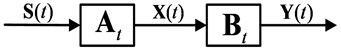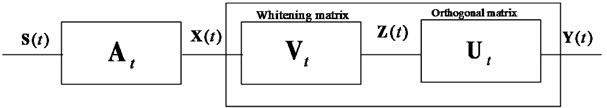### 2.2. Model assumptions of adaptive BSS

BSS problems have multiple solutions . To identify the optimal solution, five basic assumptions are proposed, which are slightly different from those used in traditional BSS :

1) ${\mathbf{A}}_{t}\in {R}^{m×k}$ satisfies $rank\left({\mathbf{A}}_{t}\right)=k$.

2) $\stackrel{\to }{s}\left(t\right)$ is a nonstationary random process.

3) $\stackrel{\to }{s}\left(t\right)$ is statistically independent at each time, and at most one component in $\mathbf{S}\left(t\right)$ obeys a Gaussian distribution.

4) The number of observed signals $\mathbf{X}\left(t\right)$ is greater than or equal to the number of source signals $\mathbf{S}\left(t\right)$.

5) $\mathbf{S}\left(t\right)$ is linearly mixed and the system is slowly time-varying.

Table 1 summarizes the differences between adaptive BSS and traditional BSS in terms of the source signals and mixing matrix.

 Problem Source signal Mixing matrix Processing requirement Tradition BSS Stationary Time invariant Offline and batch processing Adaptive BSS Non-stationary Time-varying Online and real time

## 3. Theoretical derivation of linear time-varying mixed BSS using EASI

### 3.1. Notion of equivariance

The EASI algorithm was first proposed by Cardoso, who used the notion of equivariance to prove that the performance of EASI is independent of the mixing matrix  when the mixing matrix is constant. In the same way, a blind estimate of ${\mathbf{A}}_{t}$ is simply a function of $\mathbf{X}\left(t\right)$. This can be expressed as:

4
${\stackrel{^}{\mathbf{A}}}_{t}=\mathbf{F}\left(\mathbf{X}\left(t\right)\right),$

where ${\stackrel{^}{\mathbf{A}}}_{t}$ is the estimator of ${\mathbf{A}}_{t}$ and $\mathbf{F}\left(\cdot \right)$ represents this functional relationship. In equivariance theory, when the data conversion is equivalent to some parameter conversion, both ${\stackrel{^}{\mathbf{A}}}_{t}$ and $\mathbf{X}\left(t\right)$ are multiplied on the left by a matrix $\mathbf{M}\in {R}^{k×k}$. In this case, ${\stackrel{^}{\mathbf{A}}}_{t}$ is equivariant when it satisfies:

5
$\mathbf{F}\left(\mathbf{M}\mathbf{X}\left(t\right)\right)=\mathbf{M}\mathbf{F}\left(\mathbf{X}\left(t\right)\right),$
6
$\begin{array}{l}\stackrel{^}{\mathbf{S}}\left(t\right)=\left(\mathbf{F}\left(\mathbf{X}\left(t\right)\right){\right)}^{-1}\mathbf{X}\left(t\right)=\left(\mathbf{F}\left({\mathbf{A}}_{t}\mathbf{S}\left(t\right)\right){\right)}^{-1}{\mathbf{A}}_{t}\mathbf{S}\left(t\right)=\left({\mathbf{A}}_{t}\mathbf{F}\left(\mathbf{S}\left(t\right)\right){\right)}^{-1}{\mathbf{A}}_{t}\mathbf{S}\left(t\right)\\ {\mathrm{}\mathrm{}\mathrm{}\mathrm{}\mathrm{}\mathrm{}\mathrm{}\mathrm{}\mathrm{}=\mathbf{F}\left(\mathbf{S}\left(t\right){\right)}^{-1}·\mathbf{A}}_{t}^{-1}{\mathbf{A}}_{t}\mathbf{S}\left(t\right)=\mathbf{F}\left(\mathbf{S}\left(t\right){\right)}^{-1}\mathbf{S}\left(t\right).\end{array}$

Eq. (6) shows that $\stackrel{^}{\mathbf{S}}\left(t\right)$ is given by $\stackrel{^}{\mathbf{S}}\left(t\right)=\mathbf{F}\left(\mathbf{S}\left(t\right){\right)}^{-1}\mathbf{S}\left(t\right)$, which means that it is only related to $\mathbf{S}\left(t\right)$ and is independent of ${\mathbf{A}}_{t}$: this is also called uniform performance.

### 3.2. Serial matrix updating

The core concept of EASI is serial updating . Serial updating involves choosing a $k$×$k$ matrix-valued function $y\to H\left(y\right)$, which is used to update the separating matrix ${\mathbf{B}}_{t+1}$ according to:

7
${\mathbf{B}}_{t+1}={\mathbf{B}}_{t}-{\lambda }_{t}H\left(\stackrel{\to }{y}\left(t\right)\right){\mathbf{B}}_{t},$

where ${\lambda }_{t}$ is the learning rate and is fixed in traditional EASI algorithm. Fig. 3 illustrates this update process of separation matrix. The global system ${\mathbf{E}}_{t+1}$ can be similarly updated by:

8
${\mathbf{E}}_{t+1}={\mathbf{E}}_{t}-{\lambda }_{t}H\left({\mathbf{E}}_{t}\mathbf{S}\left(t\right)\right){\mathbf{E}}_{t}.$

Eq. (8) also shows that the overall system ${\mathbf{E}}_{t}$ is not dependent on ${\mathbf{A}}_{t}$.

Fig. 3Serial update of a matrix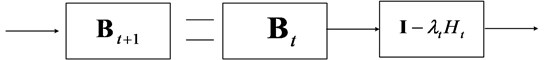### 3.3. EASI algorithm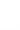Using the notion of ‘relative gradient’ , the function $H\left(y\right)$ in Eq. (7) can be considered as:

9
$H\left(\stackrel{\to }{y}\left(t\right)\right)={f}^{\mathrm{\text{'}}}\left(\stackrel{\to }{y}\left(t\right)\right){\stackrel{\to }{y}}^{T}\left(t\right).$

In Eq. (9), $f$ is an arbitrary differentiable function. Therefore, ${\mathbf{B}}_{t+1}$ can be updated according to:

10
${\mathbf{B}}_{t+1}={\mathbf{B}}_{t}-{\lambda }_{t}\left({f}^{\mathrm{\text{'}}}\left(\stackrel{\to }{y}\left(t\right)\right){\stackrel{\to }{y}}^{T}\left(t\right)-\stackrel{\to }{y}\left(t\right){f}^{\mathrm{\text{'}}}\left(\stackrel{\to }{y}\left(t\right){\right)}^{T}+\stackrel{\to }{y}\left(t\right){\stackrel{\to }{y}}^{T}\left(t\right)-\mathbf{I}\right){\mathbf{B}}_{t}.$

Let $\mathbf{I}\in {R}^{k×k}$ be the unit matrix. Exactly as in Eq. (10), according to , the serial whitening matrix ${\mathbf{V}}_{t+1}$ can be updated by:

11
${\mathbf{V}}_{t+1}={\mathbf{V}}_{t}-{\lambda }_{t}\left(\stackrel{\to }{z}\left(t\right){\stackrel{\to }{z}}^{T}\left(t\right)-\mathbf{I}\right){\mathbf{V}}_{t},$

where $\stackrel{\to }{y}\left(t\right)={\mathbf{U}}_{t}\stackrel{\to }{z}\left(t\right)$ and $\stackrel{\to }{z}\left(t\right)={\mathbf{V}}_{t}\stackrel{\to }{x}\left(t\right)$ is the signal after whitening. The orthogonal matrix ${\mathbf{U}}_{t+1}$ can also be updated by:

12
${\mathbf{U}}_{t+1}={\mathbf{U}}_{t}-{\lambda }_{t}\left({f}^{\mathrm{\text{'}}}\left(\stackrel{\to }{y}\left(t\right)\right){\stackrel{\to }{y}}^{T}\left(t\right)-\stackrel{\to }{y}\left(t\right){f}^{\mathrm{\text{'}}}\left(\stackrel{\to }{y}\left(t\right){\right)}^{T}\right){\mathbf{U}}_{t}.$

The purpose of Eq. (11) and Eq. (12) is to obtain the updated ${\mathbf{B}}_{t+1}$. Then, according to Eq. (11), Eq. (12), and ${\mathbf{B}}_{t}={\mathbf{U}}_{t}{\mathbf{V}}_{t}$, we have:

13
${\mathbf{B}}_{t+1}={\mathbf{U}}_{t+1}{\mathbf{V}}_{t+1}={\mathbf{B}}_{t}-{\lambda }_{t}\left(f\mathrm{\text{'}}\left(\stackrel{\to }{y}\left(t\right)\right){\stackrel{\to }{y}}^{T}\left(t\right)-\stackrel{\to }{y}\left(t\right)f\mathrm{\text{'}}\left(\stackrel{\to }{y}\left(t\right){\right)}^{T}+\stackrel{\to }{y}\left(t\right){\stackrel{\to }{y}}^{T}\left(t\right)$
$\mathrm{}\mathrm{}\mathrm{}\mathrm{}\mathrm{}-\mathbf{I}+O\left({\lambda }_{t}^{2}\right)\right){\mathbf{B}}_{t}\approx {\mathbf{B}}_{t}-{\lambda }_{t}\left({f}^{\mathrm{\text{'}}}\left(\stackrel{\to }{y}\left(t\right)\right){\stackrel{\to }{y}}^{T}\left(t\right)-\stackrel{\to }{y}\left(t\right){f}^{\mathrm{\text{'}}}\left(\stackrel{\to }{y}\left(t\right){\right)}^{T}+\stackrel{\to }{y}\left(t\right){\stackrel{\to }{y}}^{T}\left(t\right)-\mathbf{I}\right){\mathbf{B}}_{t}.$

In this equation, $E\left(f\mathrm{\text{'}}\left(\stackrel{\to }{y}\left(t\right)\right){\stackrel{\to }{y}}^{T}\left(t\right)-\stackrel{\to }{y}\left(t\right)f\mathrm{\text{'}}\left(\stackrel{\to }{y}\left(t\right){\right)}^{T}+\stackrel{\to }{y}\left(t\right){\stackrel{\to }{y}}^{T}\left(t\right)-\mathbf{I}\right)=$ 0, and so for $i\ne j$, $\stackrel{\to }{y}\left(i\right)$ and $\stackrel{\to }{y}\left(j\right)$ are independent of each other. For $k$ arbitrary nonlinear functions $g\left(y\right)$, we define:

14
$\stackrel{\to }{g}\left(\stackrel{\to }{y}\left(t\right)\right)=\left[{g}_{1}\left({y}_{1}\left(t\right)\right),\cdots ,{g}_{k}\left({y}_{k}\left(t\right)\right)\right],$
15
$H\left(\stackrel{\to }{y}\left(t\right)\right)=\stackrel{\to }{g}\left(\stackrel{\to }{y}\left(t\right)\right){\stackrel{\to }{y}}^{T}\left(t\right)-\stackrel{\to }{y}\left(t\right)\stackrel{\to }{g}\left(\stackrel{\to }{y}\left(t\right){\right)}^{T}.$

The EASI algorithm for adaptive source separation is based on Eq. (7) and Eq. (15):

16
${\mathbf{B}}_{t+1}={\mathbf{B}}_{t}-{\lambda }_{t}\left[\stackrel{\to }{y}\left(t\right){\stackrel{\to }{y}}^{T}\left(t\right)-\mathbf{I}+\stackrel{\to }{g}\left(\stackrel{\to }{y}\left(t\right)\right){\stackrel{\to }{y}}^{T}\left(t\right)-\stackrel{\to }{y}\left(t\right)\stackrel{\to }{g}\left(\stackrel{\to }{y}\left(t\right){\right)}^{T}]{\mathbf{B}}_{t}.$

To maintain uniform performance, which means that ${\mathbf{B}}_{t}$ can take any values, we adjust $H\left(\stackrel{\to }{y}\left(t\right)\right)$ to preserve stability. Eq. (17) describes the normalized form :

17
${\mathbf{B}}_{t+1}={\mathbf{B}}_{t}-{\lambda }_{t}\left[\frac{\stackrel{\to }{y}\left(t\right){\stackrel{\to }{y}}^{T}\left(t\right)-\mathbf{I}}{1+{\lambda }_{t}\stackrel{\to }{y}\left(t\right){\stackrel{\to }{y}}^{T}\left(t\right)}+\frac{\stackrel{\to }{g}\left(\stackrel{\to }{y}\left(t\right)\right){\stackrel{\to }{y}}^{T}\left(t\right)-\stackrel{\to }{y}\left(t\right)\stackrel{\to }{g}\left(\stackrel{\to }{y}\left(t\right){\right)}^{T}}{1+{\lambda }_{t}\left|{\stackrel{\to }{y}}^{T}\left(t\right)\stackrel{\to }{g}\left(\stackrel{\to }{y}\left(t\right)\right)\right|}\right]{\mathbf{B}}_{t}.$

### 3.4. Advantages of EASI algorithm

EASI is a gradient-based algorithm that uses nonlinear decorrelation to estimate the independent components. It has several advantages over other ICA algorithms .

1) EASI is an adaptive and online algorithm, which makes it suitable for problems in which the underlying distributions of input characteristics vary over time.

2) EASI is equivariant, and the convergence speeds, interference suppression levels, and other properties are only related to the signals’ normalized distributions and are independent of the mixing matrix.

3) EASI offers improved parallelism by combining whitening with separation, because other methods whiten the input features during a preprocessing step.

4) EASI is computationally efficient, as its basic operations only require addition and multiplication.

### 3.5. Variable learning rate EASI algorithm

In the EASI algorithm, the learning rate is closely related to the convergence speed and steady-state error. When the learning rate is fixed, values that are too large will make the algorithm unstable, whereas values that are too small will increase the convergence time .

Given the limitations of a fixed learning rate, we use a typical time-descending learning rate given by [31, 32]:

18
${\lambda }_{t}=\left\{\begin{array}{l}{\lambda }_{0},\mathrm{}\mathrm{}\mathrm{}t\le {t}_{0},\\ {\lambda }_{0}{e}^{-{t}_{d}\left(t-{t}_{0}\right)},\end{array}\right\$

where ${\lambda }_{0}$, ${t}_{0}$, and ${t}_{d}$ are constants.

### 3.6. Scope and conditions of variable learning rate EASI algorithm

1) The sources are statistically independent.

2) The source signals are non-stationary and the mixing matrix represents slow instantaneous linear mixing.

3) The number of observed signals is greater than or equal to the number of source signals, and the number of signals cannot be changed.

## 4. Simulation verification

### 4.1. Simulation dataset and parameter settings

We design simulations to demonstrate the performance of our variable learning rate EASI-based adaptive BSS method. We choose three different signals, a nonstationary wave ${\stackrel{\to }{s}}_{1}\left(t\right)$, square wave ${\stackrel{\to }{s}}_{2}\left(t\right)$, and a triangular wave ${\stackrel{\to }{s}}_{3}\left(t\right)$. These are specified as follows:

19
${\stackrel{\to }{s}}_{1}\left(t\right)=3{e}^{-0.002t}\mathrm{s}\mathrm{i}\mathrm{n}\left(7t\right),$
20
${\stackrel{\to }{s}}_{2}\left(t\right)=2\mathrm{s}\mathrm{q}\mathrm{u}\mathrm{a}\mathrm{r}\mathrm{e}\left(100t,50\right),$
21
${\stackrel{\to }{s}}_{3}\left(t\right)=2\left[a,a,a,a,a,a,a,a\right],\mathrm{}\mathrm{}\mathrm{}\mathrm{}a=\mathrm{l}\mathrm{i}\mathrm{n}\mathrm{s}\mathrm{p}\mathrm{a}\mathrm{c}\mathrm{e}\left(-1.1.25\mathrm{*}10\right).$

${\stackrel{\to }{s}}_{1}\left(t\right)$, ${\stackrel{\to }{s}}_{2}\left(t\right)$, and ${\stackrel{\to }{s}}_{3}\left(t\right)$ are independent of each other. For ${\stackrel{\to }{s}}_{1}\left(t\right)$, which is not periodic, we calculate the expectation at intervals [1, 400 s] and [201, 600 s] to be 0.0118 and 0.0082 and the mean square root to be 1.4982 and 1.0013, respectively. The expectation and mean square root change with time. Therefore, ${\stackrel{\to }{s}}_{1}\left(t\right)$ has the property of non-stationarity. The linear instantaneous mixing matrix ${\mathbf{A}}_{t}\in {R}^{3×3}$ is slowly time-varying. We use MATLAB’s “rand” function to randomly generate ${\mathbf{A}}_{t}$ as follows:

22
${\mathbf{A}}_{1}=\left[\begin{array}{lll}0.9158& 0.8975& 0.5831\\ 0.1355& 0.4996& 0.6983\\ 0.3321& 0.6153& 0.0293\end{array}\right].$

Then, we allow the first element of ${\mathbf{A}}_{t}$ to vary slowly:

23
${\mathbf{A}}_{t}\left(1,1\right)={\mathbf{A}}_{1}\left(1,1{\right)}^{\left(-0.0033t\right)}.$

Therefore, we have designed nonstationary sources and a slowly time-varying mixing matrix. The nonlinear functions $\stackrel{\to }{g}\left(\stackrel{\to }{y}\left(t\right)\right)$ are related to the signal distributions. When $\stackrel{\to }{y}\left(t\right)$ is a sup-Gaussian signal, the choice may be $\stackrel{\to }{g}\left(\stackrel{\to }{y}\left(t\right)\right)=\stackrel{\to }{y}\left(t{\right)}^{3}$. When $\stackrel{\to }{y}\left(t\right)$ is a super-Gaussian signal, our choice is usually $\stackrel{\to }{g}\left(\stackrel{\to }{y}\left(t\right)\right)=\mathrm{t}\mathrm{a}\mathrm{n}\mathrm{h}\left(\stackrel{\to }{y}\left(t\right)\right)$ . In this paper, $\stackrel{\to }{g}\left(\stackrel{\to }{y}\left(t\right)\right)=\stackrel{\to }{y}\left(t{\right)}^{3}$. The sampling length $L=$ 2000 s.

For comparison, we designed a fixed learning rate EASI, RLS method, and variable learning rate EASI. For the fixed learning rate algorithm, after a lot of experiments, we set the EASI learning rate to 0.0017 and the RLS learning rate to 0.982. For the variable learning rate parameters, we set ${\lambda }_{0}=$ 0.014, ${t}_{0}=$ 200, and ${t}_{d}=$ 0.025.

### 4.2.1. Similarity coefficient

We wish to consider the similarity, and completely eliminate the uncertainty of the order and amplitude of the output components. Most existing algorithms use the performance index (PI) as an evaluation metric, representing the closeness between ${\mathbf{B}}_{t}^{-1}$ and the mixing matrix ${\mathbf{A}}_{t}$ . The similarity coefficient between the $i$th separated signal source ${\stackrel{\to }{y}}_{i}\left(t\right)$ after normalization and the $j$th real random fault source ${\stackrel{\to }{s}}_{j}\left(t\right)$ is given by:

24
${\varsigma }_{i,j}=\frac{\left|\sum _{t=1}^{L}{{\stackrel{\to }{y}}_{i}}^{T}\left(t\right){\stackrel{\to }{s}}_{j}\left(t\right)\right|}{\sqrt{\sum _{t=1}^{L}\left({{\stackrel{\to }{y}}_{i}}^{2}\left(t\right){\right)}^{T}{\stackrel{\to }{s}}_{j}^{2}\left(t\right)}}\in \left(0,1\right),$

when the only difference in ${\stackrel{\to }{y}}_{i}\left(t\right)$ and ${\stackrel{\to }{s}}_{j}\left(t\right)$ is in their amplitudes, ${\varsigma }_{i,j}=$ 1. When ${\stackrel{\to }{y}}_{i}\left(t\right)$ is independent of ${\stackrel{\to }{s}}_{j}\left(t\right)$, ${\varsigma }_{i,j}=$ 0. Therefore, we expect to achieve values close to 1. The similarity coefficient between the $i$th separated signal source ${\stackrel{\to }{y}}_{i}\left(t\right)$ and the $j$th separated signal source ${\stackrel{\to }{y}}_{j}\left(t\right)$ after normalization is defined as:

25
${\xi }_{i,j}=\frac{\left|\sum _{t=1}^{L}{{\stackrel{\to }{y}}_{i}}^{T}\left(t\right){\stackrel{\to }{y}}_{j}\left(t\right)\right|}{\sqrt{\sum _{t=1}^{L}\left({{\stackrel{\to }{y}}_{i}}^{2}\left(t\right){\right)}^{T}\left({{\stackrel{\to }{y}}_{j}}^{2}\left(t\right){\right)}^{T}}}\in \left(0,1\right),$

when ${\stackrel{\to }{y}}_{i}\left(t\right)$ is independent of ${\stackrel{\to }{y}}_{j}\left(t\right)$, ${\xi }_{i,j}=$ 0. We therefore hope to achieve values close to 0.

The Vestigial Quadratic Mismatch (VQM) between the separated signals and the real random fault source can be used as a performance index . This metric is calculated as:

26
$\mathrm{V}\mathrm{Q}\mathrm{M}=10\mathrm{l}\mathrm{g}\left\{\frac{E\left[{\left|\stackrel{\to }{y}\left(t\right)-r{\stackrel{\to }{s}}_{i}\left(t\right)\right|}^{2}\right]}{E\left[{\left|r{\stackrel{\to }{s}}_{i}\left(t\right)\right|}^{2}\right]}\right\},$

where:

27
$r=\frac{E\left[{\stackrel{\to }{y}}_{i}\left(t\right){\stackrel{\to }{s}}_{i}^{T}\left(t\right)\right]}{E\left[{\stackrel{\to }{s}}_{i}\left(t\right){\stackrel{\to }{s}}_{i}^{T}\left(t\right)\right]},$

when the value of VQM is less than −23 dB, the effect of adaptive BSS can be considered to be very good.

### 4.3. Simulation results

The source signals used in this simulation are shown in Fig. 4. From top to bottom, they are a non-stationary wave ${\stackrel{\to }{s}}_{1}\left(t\right)$, a square wave ${\stackrel{\to }{s}}_{2}\left(t\right)$, and a triangular wave ${\stackrel{\to }{s}}_{3}\left(t\right)$. After linear instantaneous mixing, the observed signals are shown in Fig. 5. Figs. 6-9 show the separation results of the fixed learning rate EASI, the fixed learning rate RLS, and the variable learning rate EASI method.

Fig. 4Three different nonstationary source signals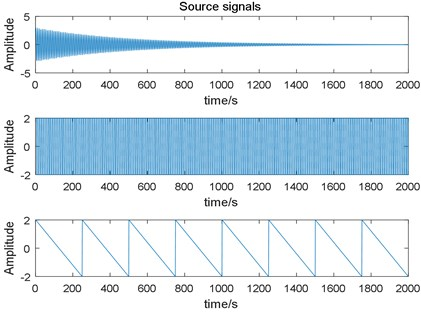Fig. 5Signals after linear mixing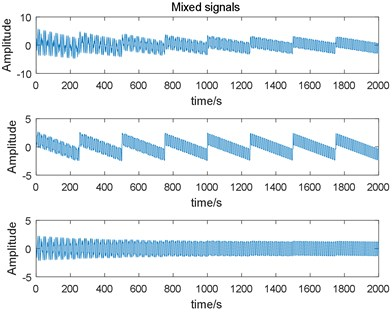Fig. 6Estimated signals output by the fixed learning rate EASI algorithm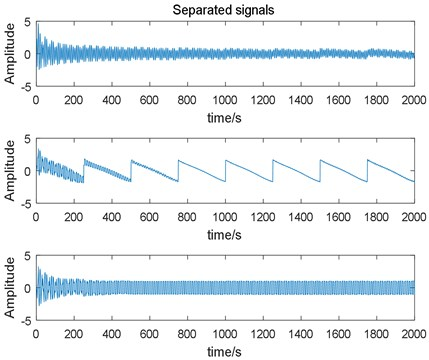Fig. 7Estimated signals output by the fixed learning rate RLS algorithm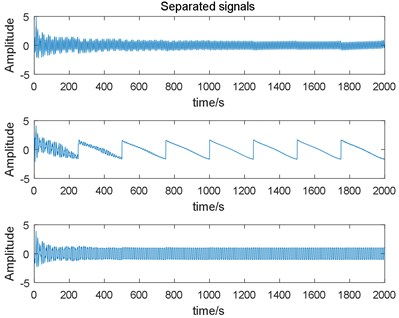Fig. 8Estimated signals output by the variable learning rate EASI algorithm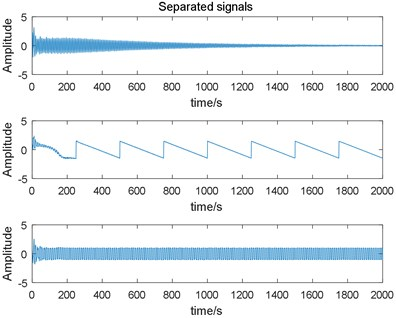Table 2 presents the separation results achieved by EASI, RLS, and variable learning rate EASI. Table 3 demonstrates the independence of the source signals. Table 4 lists the similarity between the separated signals given by EASI, RLS, and variable learning rate EASI.

Fig. 9 shows the variation of the first element of the mixing matrix over time.

Table 2Separation results of three methods

 Method Corresponding relationship Similarity coefficient VQM EASI ${\stackrel{\to }{y}}_{1}\left(t\right)$ responding to ${\stackrel{\to }{s}}_{1}\left(t\right)$ 0.8233 –3.2300 ${\stackrel{\to }{y}}_{2}\left(t\right)$ responding to ${\stackrel{\to }{s}}_{3}\left(t\right)$ 0.9446 –9.0979 ${\stackrel{\to }{y}}_{3}\left(t\right)$ responding to ${\stackrel{\to }{s}}_{2}\left(t\right)$ 0.9799 –13.8283 RLS ${\stackrel{\to }{y}}_{1}\left(t\right)$ responding to ${\stackrel{\to }{s}}_{1}\left(t\right)$ 0.8066 –2.6945 ${\stackrel{\to }{y}}_{2}\left(t\right)$ responding to ${\stackrel{\to }{s}}_{3}\left(t\right)$ 0.9446 –9.0652 ${\stackrel{\to }{y}}_{3}\left(t\right)$ responding to ${\stackrel{\to }{s}}_{2}\left(t\right)$ 0.9863 –15.5217 Variable learning rate EASI ${\stackrel{\to }{y}}_{1}\left(t\right)$ responding to ${\stackrel{\to }{s}}_{1}\left(t\right)$ 0.9575 –10.4256 ${\stackrel{\to }{y}}_{2}\left(t\right)$ responding to ${\stackrel{\to }{s}}_{3}\left(t\right)$ 0.9845 –14.8274 ${\stackrel{\to }{y}}_{3}\left(t\right)$ responding to ${\stackrel{\to }{s}}_{2}\left(t\right)$ 0.9911 –21.5032

Table 3Similarity and independence between source signals

 Corresponding relation between source signals Similarity coefficient between source signals $P$-value of Chi-square test ${\stackrel{\to }{s}}_{1}\left(t\right)$ responding to ${\stackrel{\to }{s}}_{2}\left(t\right)$ 0.0017 0.4895 ${\stackrel{\to }{s}}_{1}\left(t\right)$ responding to ${\stackrel{\to }{s}}_{3}\left(t\right)$ –0.0027 0.4012 ${\stackrel{\to }{s}}_{2}\left(t\right)$ responding to ${\stackrel{\to }{s}}_{3}\left(t\right)$ 0.0013 1

Table 4Similarity between separation signals

 Method Corresponding relationship Similarity coefficient EASI ${\stackrel{\to }{y}}_{1}\left(t\right)$ responding to ${\stackrel{\to }{y}}_{2}\left(t\right)$ 0.2383 ${\stackrel{\to }{y}}_{1}\left(t\right)$ responding to ${\stackrel{\to }{y}}_{3}\left(t\right)$ 0.3525 ${\stackrel{\to }{y}}_{2}\left(t\right)$ responding to ${\stackrel{\to }{y}}_{3}\left(t\right)$ 0.1662 RLS ${\stackrel{\to }{y}}_{1}\left(t\right)$ responding to ${\stackrel{\to }{y}}_{2}\left(t\right)$ 0.0982 ${\stackrel{\to }{y}}_{1}\left(t\right)$ responding to ${\stackrel{\to }{y}}_{3}\left(t\right)$ 0.2144 ${\stackrel{\to }{y}}_{2}\left(t\right)$ responding to ${\stackrel{\to }{y}}_{3}\left(t\right)$ 0.1557 Variable learning rate EASI ${\stackrel{\to }{y}}_{1}\left(t\right)$ responding to ${\stackrel{\to }{y}}_{2}\left(t\right)$ 0.0531 ${\stackrel{\to }{y}}_{1}\left(t\right)$ responding to ${\stackrel{\to }{y}}_{3}\left(t\right)$ 0.0172 ${\stackrel{\to }{y}}_{2}\left(t\right)$ responding to ${\stackrel{\to }{y}}_{3}\left(t\right)$ 0.0447

Fig. 9The variation of A1(1,1)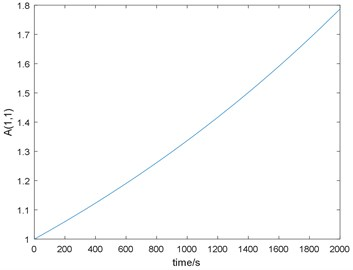### 4.4. Simulation results analysis

The simulation results can be analyzed as follows:

1) The first separation signal in Fig. 6 corresponds to the first source signal in Fig. 4, the second separation signal ${\stackrel{\to }{y}}_{2}\left(t\right)$ corresponds to the third source signal ${\stackrel{\to }{s}}_{3}\left(t\right)$, and ${\stackrel{\to }{y}}_{3}\left(t\right)$ corresponds to ${\stackrel{\to }{s}}_{2}\left(t\right)$. The sequence of the separated signals is uncertain, which is a problem with the adaptive BSS algorithm.

2) From Fig. 4, we can see that ${\stackrel{\to }{s}}_{1}\left(t\right)$ is nonstationary. In Table 3, if the $p$-value is close to 0, the source signals are not independent. Therefore, the sources are nonstationary and independent, and can be used to verity the algorithm.

3) The shapes of the identified signals are similar to those of the source signals (see Fig. 4 and Figs. 6-8) in the different methods. This result is confirmed by Table 2. The fixed learning rate EASI and RLS are not as good as variable learning rate EASI in the case of nonstationary environments and time-varying mixing.

4) In Table 4, the similarity coefficient between separated signals is less than 6 % in variable learning rate EASI, demonstrating that the correlation between the separated signals is not high and the separation is very good.

5) Fig. 9 illustrates the exponential increase of ${\mathbf{A}}_{1}\left(1,1\right)$. Though ${\mathbf{A}}_{1}$ was chosen at random, the selection of the initial value ${\mathbf{A}}_{1}\left(1,1\right)$ has a significant influence on the simulation results. The reason for this requires further study.

6) In Tables 2 and 4, the variable learning rate has a significant impact on the results. The variable learning rate EASI achieves better results than the other algorithms, and RLS outperforms EASI. The separation signals obtained by all methods are similar to the source signals, and the correlation between the separated signals is not high.

7) Similarity coefficients and VQM can both be used to evaluate the separation results. In Table 2, when the similarity coefficients are the same, VQM can still distinguish the results.

## 5. Conclusions

In this paper, we have described a variable learning rate EASI algorithm for adaptive BSS with a nonstationary source and slowly time-varying environment. This algorithm achieves good accuracy in terms of source separation.

However, the accuracy of the algorithm is closely related to the choice of parameters. The current learning rate and initial value are selected in advance based on experience, rather than using the degree of non-stationarity of the system response signal. In future work, we will identify more complex time-varying situations, such as changing the number of sources or the mixing matrix dimensions, explore the effects of parameter values on the results, and present experimental verifications of actual scenarios.

02 June 2018
Accepted
26 December 2018
Published
15 May 2019
SUBJECTS
Fault diagnosis based on vibration signal analysis
Keywords
equivariant adaptive source separation via independence
variable learning rate
nonstationary